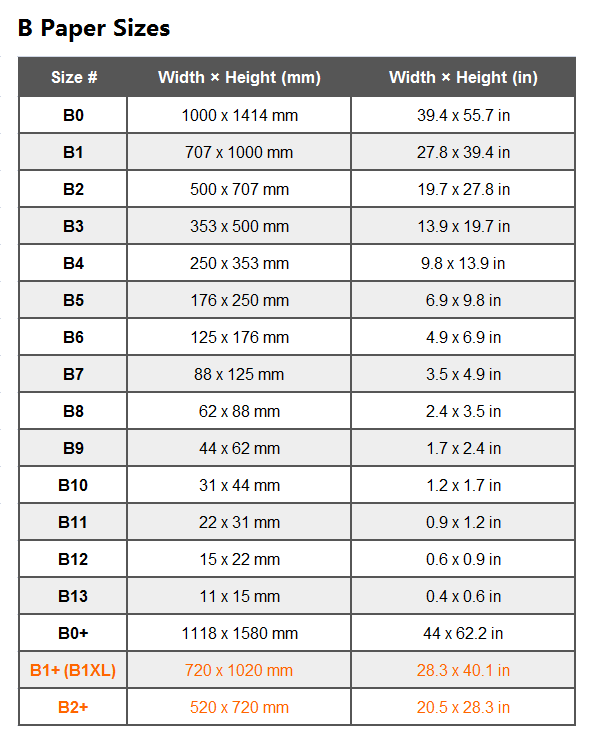# B Paper Sizes

Similar to the A paper sizes, the size of B series paper continues the definition of ISO216 international paper size standard, that is, the ratio of length to width is √2:1. Also the naming follows the same way as A series, starting from 0 and continuing after 10. For example, B0, B1, B2, B3, B4, B5, B6, B7, B8, B9, B10 ..

## Sizes Rules

There are similar rules of B series sizes as of A paper sizes, defined by ISO216:

• Named from B0 to B10 and even after: B0, B1, B2, B3, B4, B5, B6, B7, B8, B9, B10..
• Width(B0) =  Exactly 1 meter
• The length of the sheet is equal to its width times 1.4142 (√2:1).
• Bn is devided into two equal parts of B(n-1) size, so
• S(Bn) = 2 x S[B(n-1)]  (S means total area)

## B Paper Size Chart |Dimension Table of B Series Paper Sizes

Here is the paper sizes chart of B series, in which you can find the dimensions of each size # in mm and inches. How to get the dimension in centimeters- simply divide the data value in millimeters by 10, and then it will be the dimension in cm.B Paper Sizes Chart: Dimension of B0, B1, B2, B3, B4, B5, B6, B7, B8, B9, B10, B11, B12, B13 paper and more

#### Exceptions: B1+ (B1XL) and B2+

The B1+ 1050 mm × 750 mm (41.3 in × 29.5 in) also named B1XL, and B2 + 750 mm × 530 mm (29.5 in × 20.9 in) are both uncut and oversize of B sizes. They are not defined by SO216 standard, because they do not follow the √2:1 size ratio. B1+ . These kind of size appear more for the special needs of the printing and related industries. We list here to make it clear that there are some special sizes, named by the sequence B (or similar), but not actually in the ISO216 series.

## Usage of B Series Pape Sizes

B series paper sizes are not as widely used as A series sizes. They were introduced as special purposes, which are not common in office use and are more applied to the printing of books of various specifications, as well as posters and envelopes.

## Dimension Tolerance

Similar to the size of A series paper, due to inaccurate manual operation or low machine accuracy, in the process of cutting and manufacturing, there will always be more or less tolerances. Tolerances for B paper dimensions according to ISO216 are as follows.

• When the length and width of the paper are less than 150 mm, 5.9 inches, the tolerance is ±1.5 mm, i.e. 0.06 inches.
• When the height and width are between 150 mm and 600 mm, or 5.9 to 23.6 inches, the tolerance is ±2 mm, or 0.08 inches.
• The tolerance is ±3 mm, or 0.12 in., for both length and width greater than 600 mm and 23.6 in.

## Areas

The areas of B series paper sizes are the geometric average of consecutive the areas of A series paper sizes. For example, the area of A0 is one square meter, then the area of B0 is larger than one square meter, and times it 1.1442. That is,

• S(Bn)=S(An)x1.4142

## RB & SRB Paper Sizes

In the early days, the sizes of B paper series encountered the same problem as the sizes of A paper series, that was, in industrial printing, the paper edge ink penetration might cause the problem of insufficient size after cutting. In this case, paper sizes such as RB (Raw Format B) and SRB (Supplementary Raw Format B) were also introduced. The aim was that after cutting out the ink stained paper edges, the remaining size is exactly the standard ISO216 sizes.